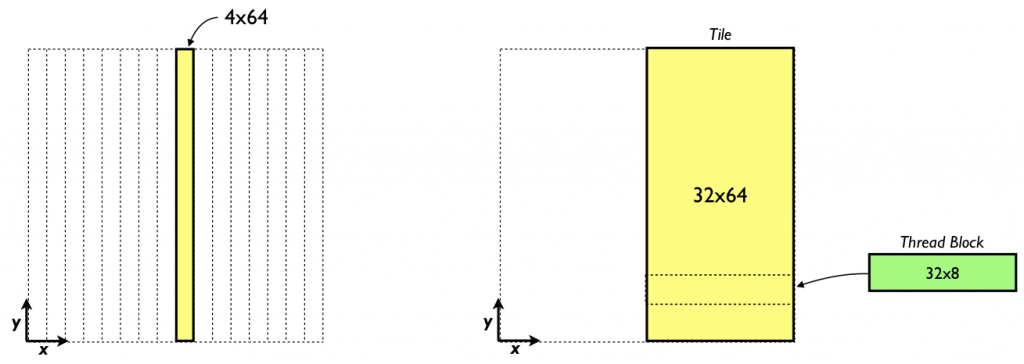# Finite Difference Methods in CUDA Fortran, Part 2CUDA Fortran for Scientists and Engineers shows how high-performance application developers can leverage the power of GPUs using Fortran.

In the last CUDA Fortran post we dove in to 3D finite difference computations in CUDA Fortran, demonstrating how to implement the derivative part of the computation. In this post, let’s continue by exploring how we can write efficient kernels for the y and derivatives. As with the previous post, code for the examples in this post is available for download on Github.

## Y and Z Derivatives

We can easily modify the derivative code to operate in the other directions. In the derivative each thread block calculates the derivatives in an x, y tile of 64 × `sPencils` elements. For the derivative we can have a thread block calculate the derivative on a tile of `sPencils × `64 elements in x, y, as depicted on the left in the figure below.Likewise, for the derivative a thread block can calculate the derivative in a x, z tile of `sPencils × `64 elements. The kernel below shows the derivative kernel using this approach.

```attributes(global) subroutine derivative_y(f, df)
implicit none
real, intent(in) :: f(mx,my,mz)
real, intent(out) :: df(mx,my,mz)
real, shared :: f_s(sPencils,-3:my+4)
integer :: i,i_l,j,k

k = blockIdx%y

f_s(i_l,j) = f(i,j,k)

if (j <= 4) then
f_s(i_l,j-4) = f_s(i_l,my+j-5)
f_s(i_l,my+j) = f_s(i_l,j+1)
endif

df(i,j,k) = &
(ay_c *( f_s(i_l,j+1) - f_s(i_l,j-1) ) &
+by_c *( f_s(i_l,j+2) - f_s(i_l,j-2) ) &
+cy_c *( f_s(i_l,j+3) - f_s(i_l,j-3) ) &
+dy_c *( f_s(i_l,j+4) - f_s(i_l,j-4) ))

end subroutine derivative_y```

By using the shared memory tile we can ensure that each element from global memory is read in only once.

`  real, shared :: f_s(sPencils,-3:my+4)`

The disadvantage of this approach is that with `sPencils` or 4 points in x for these tiles we no longer have perfect coalescing. The performance results bear this out; we achieve roughly half the performance of the derivative kernel.

```Using shared memory tile of x-y: 4x64
RMS error: 5.7687557E-06
MAX error: 2.3365021E-05
Average time (ms): 5.9840001E-02
Average Bandwidth (GB/s): 32.63912```

In terms of accuracy, we obtain the same maximum error of the derivative, but a different (not substantially worse) RMS error for essentially the same function. This difference is due to the order in which the accumulation is done on the host, simply swapping the order of the loops in the host code error calculation would produce the same results.

```attributes(global) subroutine derivative_y_lPencils(f, df)
implicit none
real, intent(in) :: f(mx,my,mz)
real, intent(out) :: df(mx,my,mz)
real, shared :: f_s(lPencils,-3:my+4)
integer :: i,j,k,i_l

k = blockIdx%y

do j = threadIdx%y, my, blockDim%y
f_s(i_l,j) = f(i,j,k)
enddo

if (j <= 4) then
f_s(i_l,j-4) = f_s(i_l,my+j-5)
f_s(i_l,my+j) = f_s(i_l,j+1)
endif

do j = threadIdx%y, my, blockDim%y
df(i,j,k) = &
(ay_c *( f_s(i_l,j+1) - f_s(i_l,j-1) ) &
+by_c *( f_s(i_l,j+2) - f_s(i_l,j-2) ) &
+cy_c *( f_s(i_l,j+3) - f_s(i_l,j-3) ) &
+dy_c *( f_s(i_l,j+4) - f_s(i_l,j-4) ))
enddo
end subroutine derivative_y_lPencils```

Here we set `lPencils` equal to 32. This code is similar to the above y derivative code except the variable `j` is a loop index rather than being calculated once.  When we use a large amount of shared memory per thread block, we should check to see the effect of whether and by how much the shared memory use limits the number of threads that reside on a multiprocessor.  Each thread block requires 9216 bytes of shared memory, and with a limit of 48 KB of shared memory per multiprocessor, this translates to a maximum of 5 thread blocks that can reside at one time on a multiprocessor.  (Compiling with `-Mcuda=ptxinfo` indicates each thread uses 20 registers which will not limit the number of thread blocks per multiprocessor.)  With a thread block of 32×8 threads, the number of threads that can reside on a multiprocessor for this kernel is 1280 versus a maximum of 1536 on the C2050.  The occupancy is 1280/1536 or 0.83, which is not a problem in general, especially since we are using eight-fold instruction-level parallelism via the loops over `j`. Here are the results for this kernel.

```Using shared memory tile of x-y: 32x64
RMS error: 5.8093055E-06
MAX error: 2.3365021E-05
Average time (ms): 3.3199999E-02
Average Bandwidth (GB/s): 58.82907```

This surpasses the bandwidth of the x derivative kernel. You might wonder if using such a larger number of pencils in the shared memory tile will improve performance of the x derivative code presented earlier. This ends up not being the case.

```Using shared memory tile of x-y: 64x32
RMS error: 5.8098590E-06
MAX error: 2.3365021E-05
Average time (ms): 3.7150402E-02
Average Bandwidth (GB/s): 52.57346```

Recall that for the x derivative we already have perfect coalescing for the case with four pencils. Since the occupancy of this kernel is high there is no benefit from the added instruction-level parallelism. As a result, the additional code to loop over portions of the shared-memory tile simply add overhead and as a result performance decreases. We handle the z derivative in the same way as the y derivative, and achieve comparable performance.

In the past several posts we have explored the use of shared memory to optimize kernel performance. The transpose example showed how shared memory can be used to help global memory coalescing, and in the finite difference code we showed how shared memory can assist with data reuse.